# ELECTRIC CURRENT

## Electricity of Class 9

The electric current is a flow of electric charges (called electrons) in a conductor. The magnitude of electric current in a conductor is the amount of electric charge passing through a given point of the conductor in one second. If a charge of Q coulombs flows through a conductor in time t seconds, then the magnitude of the electric current I flowing through it is given by: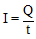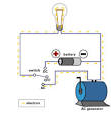The unit of charge, in S.I. system is coulomb, which is equivalent to the charge of nearly 6.25 × 1018 electrons. If charge is measured in coulomb, then the flow of 1 coulomb/second gives us the unit of current, which is called Ampere named in the honour French scientist, Andre - Marie Ampere (1775 – 1836).

### UNIT OF ELECTRIC CURRENT

SI unit of current is Ampere (A)

1 Ampere =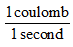Therefore, 1 ampere of current is said to be flowing through the conductor if one coulomb of charge flows through it in one second.

1 mA (milliampere) =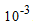A

1μA (microampere) =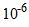A

### DIRECTION OF ELECTRIC CURRENT:

When electricity was invented, it was known that there are two types of charges : positive charges and negative charges, but the electron had not been discovered at that time. So, electric current was considered to be a flow of positive charges and the direction of flow of the positive charges was taken to be the direction of electric current. Thus, the conventional direction of electric current is from positive terminal of a cell (or battery) to the negative terminal through the circuit.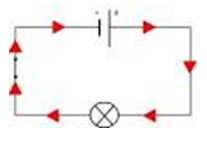Figure showing direction of flow of conventional current

Ben Franklin, who conducted extensive scientific studies in both static and current electricity, envisioned positive charges as the carriers of charge. As such, an early convention for the direction of an electric current was established to be in the direction that positive charges would move. The convention has stuck and is still used today. The direction of an electric current is by convention the direction in which a positive charge would move. Thus, the current in the external circuit is directed away from the positive terminal and toward the negative terminal of the battery. Electrons would actually move through the wires in the opposite direction. Knowing that the actual charge carriers in wires are negatively charged electrons may make this convention seem a bit odd and outdated. Nonetheless, it is the convention that is used worldwide and one that a student of physics can easily become accustomed to.

### HOW THE CURRENT FLOWS IN A WIRE :

As electric current is the flow of electrons in a metal wire (or conductor) when a cell or battery is connected across its ends. A metal wire has plenty of free electrons in it. When the metal wire has not been connected to a source of electricity like a cell or a battery, then the electrons present in it move at random in all the directions between the atoms of the metal wire as shown in figure.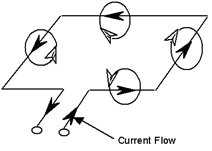When a source of electricity like a cell or a battery is connected between the ends of the metal wire, then an electric force acts on the electrons present in the wire. Since the electrons are negatively charged, they start moving from negative end to the positive end of the wire and this flow of electrons constitutes the electric current in the wire.

### HOW TO GET A CONTINUOUS FLOW OF ELECTRIC CURRENT:

It is due to the potential difference between two points that an electric current flows between them. The simplest way to maintain a potential difference between the two ends of a conductor so as to get a continuous flow of current is to connect the conductor between the terminals of a cell or a battery.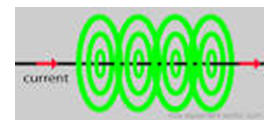Due to the chemical reactions going on inside the cell or battery, a potential difference is maintained between its terminals and this potential difference drives the current in a circuit.